# 3. Fagor logo¶

In this exercise we will use the subtraction Boolean operation to draw the proposed logo.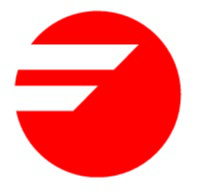1. First we open a new document with Inkscape.

2. Next we will create a circle with the draw circles and ellipses tool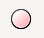.

Once the circle has been drawn, we are going to change its dimensions in the upper bar of Inkscape to 30 millimeters of horizontal radius and 30 millimeters of vertical radius.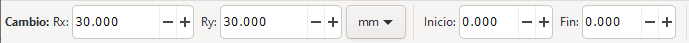With what we will obtain a circle of 30 millimeters of radius.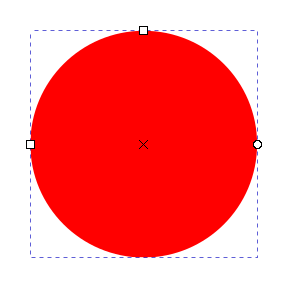3. Next we are going to draw with the squares and rectangles tool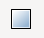a blue rectangle.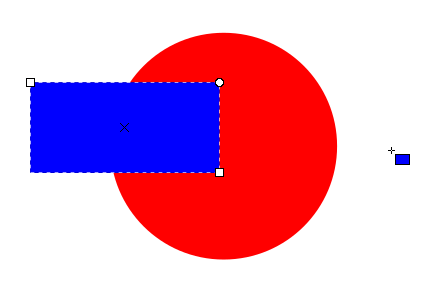Once drawn we are going to change its size to 50 millimeters wide by 24 millimeters high.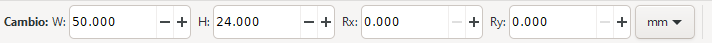4. To continue we will tilt the rectangle -45 degrees horizontally with the `Object... Transform...` menu tool. In the Skew tab we will write -45 horizontally.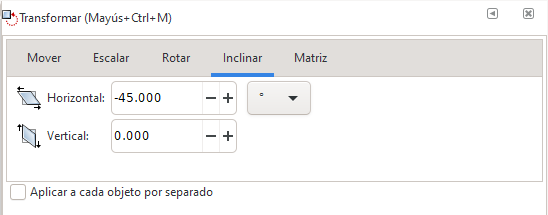When you press the Apply button, the image will look like this.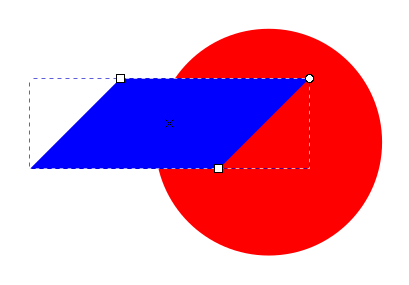5. Following the same method as before, we will draw a green rectangle 100 millimeters wide by 6 millimeters high.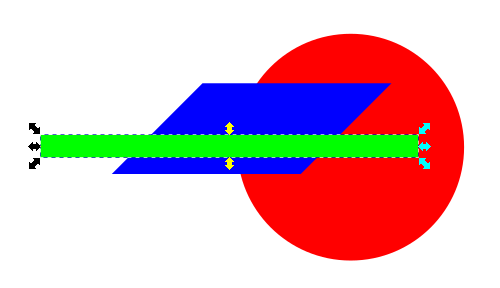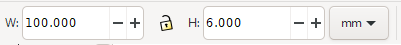6. Now we select the two rectangles and center them on the horizontal axis.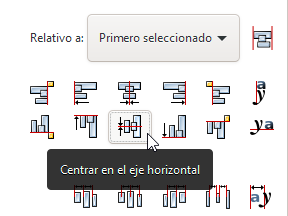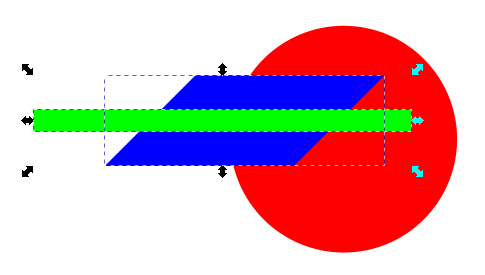7. To continue we choose in the menu `Path... Difference` with which we will obtain a split rectangle like the one in the figure.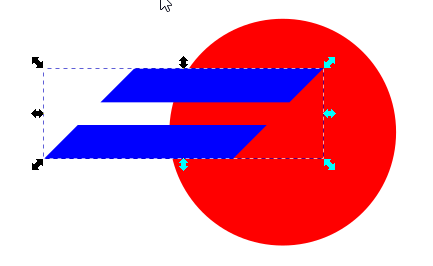8. Finally, we select all the objects and choose again from the `Path... Difference` menu to obtain the logo we are looking for.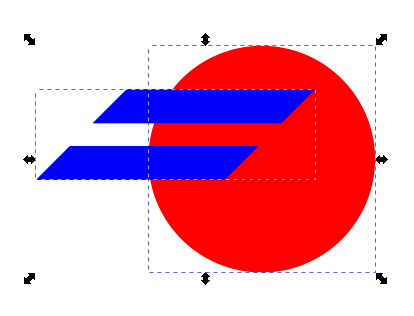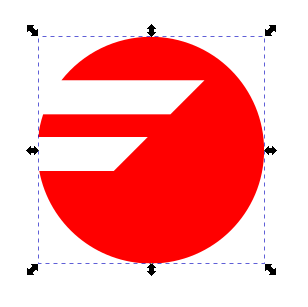9. If we now choose the node editing tool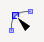We can see that the logo created is a single figure made up of a total of 11 editable nodes.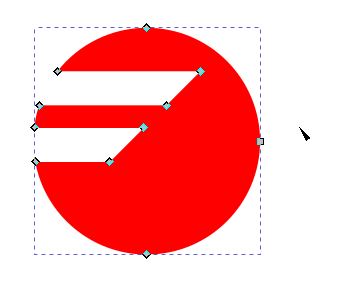10. When deselecting the figure we will see the logo created.